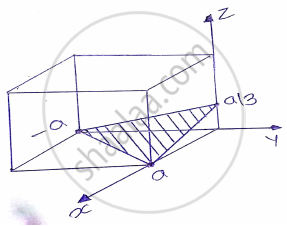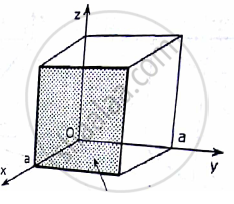# Draw the following : (1,-1,3) ,(2,0,0) , [0,0,-1]An electron is accelerated through 1200 volts and is reflected from a crystal. The second order reflection occurs when glancing angle is 60°. - Applied Physics 1

Short Note

Draw the following : (1,-1,3) ,(2,0,0) , [0,0,-1]
An electron is accelerated through 1200 volts and is reflected from a crystal. The second order reflection occurs when glancing angle is 60°. Calculate the inter planar spacing of the crystal.

#### Solution

Ans :- 1. Miller indices  :( 1 1 3)
Intercepts   :a, -a, a/32. Miller indices : (200) …………(can also be written as (100))
Reciprocals : 1, ∞ ,∞
Intercepts : a, ∞ ,∞Concept: Introduction to Crystallography
Is there an error in this question or solution?